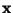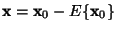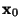Next: Second-order methods Up: Classical Linear Transformations Previous: Classical Linear Transformations

## Introduction

Several principles have been developed in statistics, neural computing, and signal processing to find a suitable linear representation of a random variable. In this Section, we discuss classical methods for determining the linear transformation as in (2). All the methods discussed in this paper are based on using centered variables. In other words, the mean of the random vector is subtracted. To simplify the discussion, it is henceforth assumed that the variableis centered, which means that it has already been transformed by, whereis the original non-centered variable.

Aapo Hyvarinen
1999-04-23